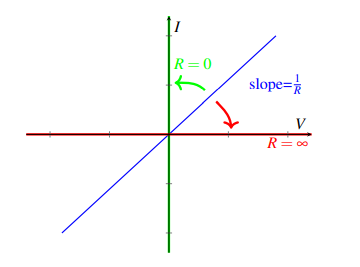# Notes can be found as interactive webpage at

7: 2D Touchscreens & Superp. + Equivalence


# 03-08: 2D Resistive Touchscreens #

## Bottom Plate #

• Trivially, we see that if we add a wire connected to $u_\text{mid}$ that will have the same voltage across it (wires have zero voltage drop)
• So why have the bottom plate at all? It lets us take the measurement for $V_\text{out}$ using connection points on the edge of the plate instead of having to put a probe or wire at the actual touch point every time!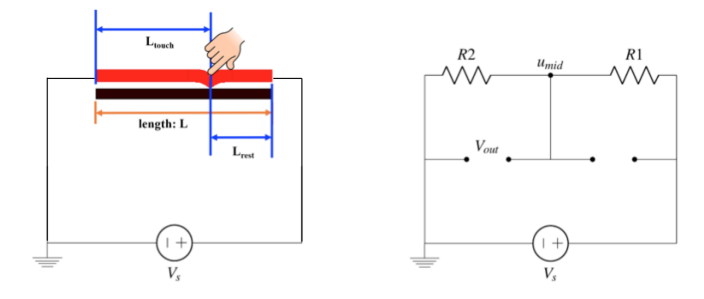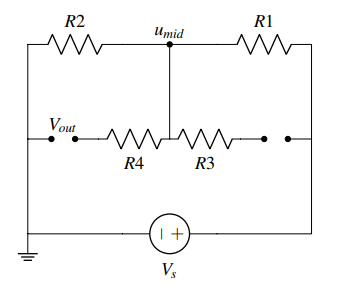### What if this bottom plate is non-ideal? That is, $R \neq 0$ ? #

• Both of these resistors are followed by an open circuit. From the definition of an open circuit, we know that zero current will flow through it.
• If $i_\text{mid} = i_{R4} +i_{R3}$ from KCL, and $i_{R4} = i_{R3} = 0$ from the definition of an open circuit (Ohm’s Law says that $0V = R \cdot 0A$), the voltage across these new resistors will be $0$.
• This means that, even with an imperfectly conductive bottom plate, the voltage $V_\text{out}$ will still be equal to $u_\text{mid}$, even with the addition of these new resistors.
• To measure an output voltage, we need to put some device at the open circuit labeled $V_\text{out}$.

## Interesting Circuit #

• These are two parallel voltage dividers, thus we can write:

$$u_2 = \frac{kR_1}{R_1 + k R_1} V_s = \frac{k}{1+k}V_S$$ $$u_3 = \frac{kR_2}{R_2 + k R_2} V_S = \frac{k}{1+k}V_S$$

• We see that regardless of the resistances $R_1$ and $R_2$, the potentials $u_2$ and $u_3$ are the same! This holds as long as $k$ is constant: $$u_2 = u_3 = \frac{k}{1+k}V_S$$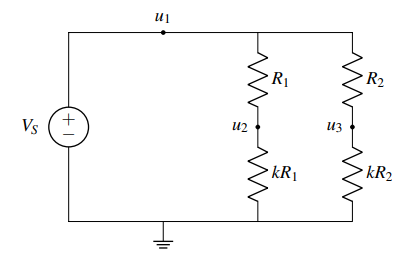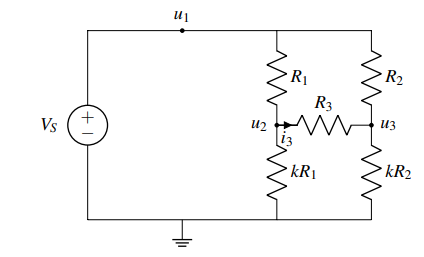• We can add $R_3$ and it won’t change the circuit behavior
• Since $u_2 = u_3$, there is no $\Delta V$, thus no current flows through the resistor.
• We can also find this with KCL: $R_3 i_3 = u_2 - u_3 = 0 \therefore i_3 = 0$
• This means that $R_3$ is at the special $(0, 0)$ point on the $I$-$V$ plot, where it behaves the same way as a wire or open circuit
• That is, we could replace $R_3$ with an open circuit and nothing would change

## 2D Resistive Touchscreen #

• Now, let’s introduce the physical structure of a 2D touchscreen: it consists of a top red plate and a bottom black plate. When a finger touches the screen, the top red plate is pushed into contact with the bottom black plate at the touch point.
• The top and bottom ends of the top red plate as well as the left and right ends of the bottom black plate are made of materials that have very low resistivities $\rho$, we can treat them as ideal wires ($\rho = 0$). The materials of the transparent screen that we touch in the middle have much higher resistivity.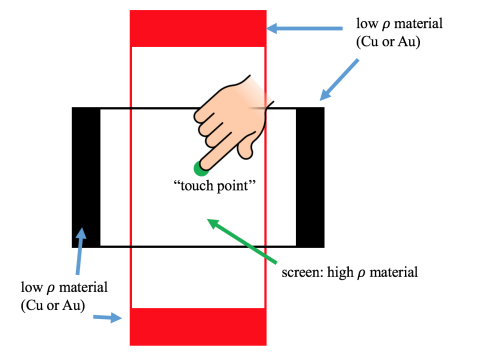### Top Plate #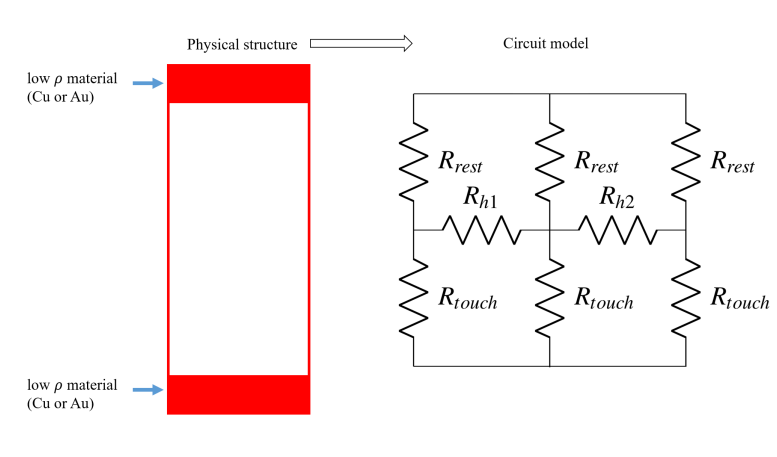• We can treat the red plate as a bunch of vertical resistor strips, where each vertical strip is connected to the strips next to it by horizontal resistors as well.
• When we touch the plate, we split it into a top and bottom half, or $R_\text{rest}$ and $R_\text{touch}$.
• Rather than considering many vertical strips, we will divide the red plate into just three equal vertical segments represented by resistors, which are connected by horizontal resistors $R_{h1}$ and $R_{h2}$.

• Adding a voltage supply $V_S$ we can see this is the same interesting circuit as before!
• Since $R_\text{rest}$ and $R_\text{touch}$. are the same for each segment, we know that $u_2 = u_3 = u_4$. As with the “interesting circuit” can replace horizontal resistors $R_{h1}, R_{h2}$ with open circuits.

$$u_3 = \frac{R_\text{touch}}{R_\text{rest} + R_\text{touch}}V_S$$ $$\dots = \frac{\rho \frac{L_\text{touch}}{A}}{\rho \frac{L_\text{touch}}{A} + \rho \frac{L_\text{rest}}{A}}$$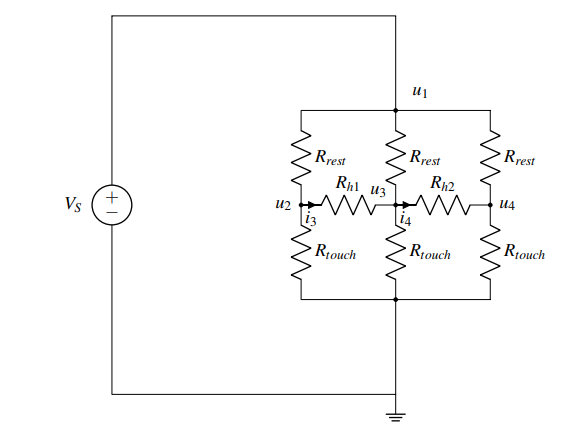$$\dots = \frac{L_\text{touch}}{L}V_S \text{ for } L_\text{touch} = L_\text{touch, vertical}$$

• This means that $u_3$ is mapped to the vertical position touched in the same way as the 1D touchscreen. When measuring the vertical position touched ($L_\text{touch, vertical}$), the bottom black plate connects to a voltmeter and measures $u_3$, the same way it did in the 1D touchscreen
• Note that, although we have represented the top red plate by three segments of equal width in the circuit model we built, the value of $u_3$ will remain the same if we choose to represent the top red plate by an infinite number of segments.

## Bottom Plate #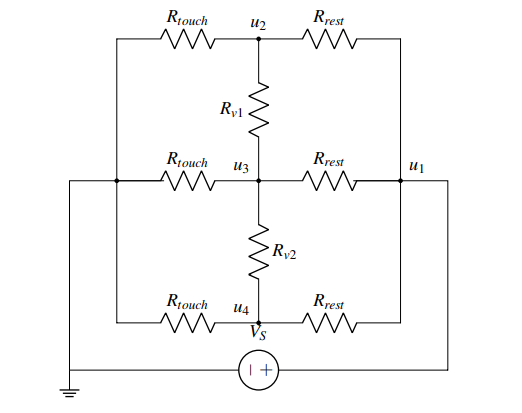• We know from linear algebra that if we want to find two values (i.e. vertical and horizontal position), we will need two measurements.
• To find the horizontal position, we connect the supply voltage source $V_S$ to the bottom black plate, and connect the top red plate to a voltmeter. As before, we choose to represent the bottom black plate by three segments of equal width which are connected in between by vertical resistors $R_{v1}, R_{v2}$.

$$u_3 = \frac{R_\text{touch}}{R_\text{touch} + R_\text{rest}} V_S = \frac{L_\text{touch}}{L}V_S$$

• The important simplification used is replacing $R_{h1}, R_{h2}$. with open circuits for the $L_\text{touch}$, horizontal measurement and $R_{v1}, R_{v2}$. for the $L_\text{touch}$, vertical measurement.
• However, this kind of simplification is valid only if the resistor is at $(0, 0)$ on the $I$-$V$ plot, which means the resistor has zero current flow and therefore zero voltage drop ($IR = V$).
• From the $I$-$V$ plots, although a resistor, a wire and an open circuit can behave quite differently, their behaviors are exactly the same at $(0, 0)$.
• This means that at $(0, 0)$, these three circuit elements can be replaced by one another and the same behavior $(I = 0, V = 0)$ is still expected.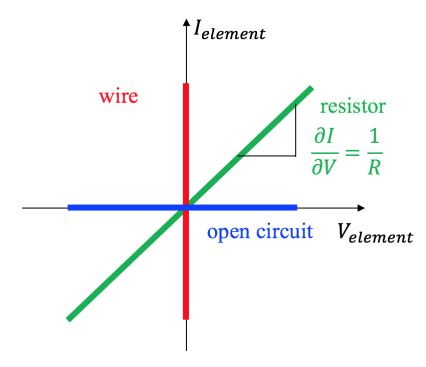## Fast Analysis #

Prior, we used the fact that all of the segments had $R_\text{rest} = R_\text{touch}$. In this section, we’ll consider a similar circuit where the resistances are all different

1. Write equations for the nodes with voltage sources between them.
• Here, the ground node and $u_1$ have $V_S$ between them, so $u_1 = V_S$
2. Write KCL for any unknown nodes, using the $V = IR$ relationship, and taking into account any current sources connected to the node
• If we consider node $u_2$, the sum of the currents flowing out of $u_2$ through $R_1, R_5, R_3$ is 0. $$\frac{u_2 - V_S}{R_1} + \frac{u_2}{R_3} + \frac{u_2 - u_3}{R_5} = 0,$$ $$\frac{u_3 - V_S}{R_2} + \frac{u_3}{R_4} + \frac{u_3 - u_2}{R_5} = 0$$
• Now, we have just two equations and two unknowns ($u_2$ and $u_3$) so all the remains to be done is to solve the equations by hand.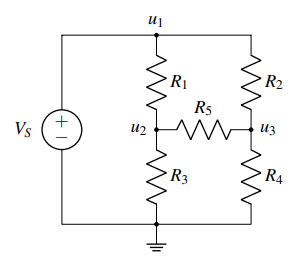You can check that the signs of your voltage drops are correct by checking that the node in question is always positive in the numerators. So in the first equation, $u_2$ is positive, while in the second equation, $u_3$ is positive

### What if we have a current source? How do we KCL? #

Remember that we treat all currents as flowing out of the node. We should also take the direction of the current source into account.

In this case, the left branch of $u_1$ has a current source $I_S$ flowing in, so $−I_S$ current flows out. So our equation is

$$-I_S + \frac{u_1 - u_2}{R_1} + \frac{u_1 - u_3}{R_2} = 0$$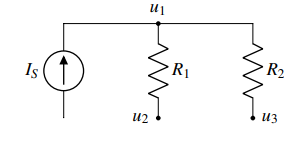# 03-10: Superposition and Equivalence #

## Equivalence #

If we pick two terminals within a circuit, we say that another circuit is equivalent to the original circuit if it exhibits the same $I$-$V$ relationship at those two terminals.

• An example of an $I$−$V$ is that of a resistor, i.e., $V = IR$ or $I= \frac{V}{R}$
• We can do this since voltage and current are governed by a linear relationship (in 16A), and a line can be uniquely determined by exactly two points, we can capture the original circuit with a simplified circuit that has exactly two components: a voltage (or current) source and a resistor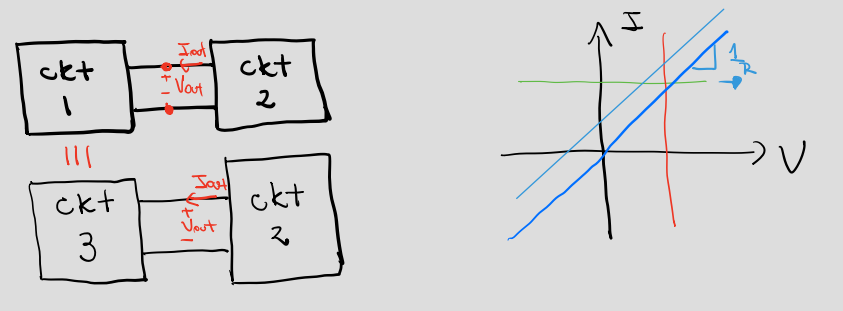### Short-circuit current: #

Current $I_\text{sc} \equiv I_{No}$ when $V_S = 0$. Used for norton equivalent

If we look at the $I$-$V$ plot of a voltage source $V_S$, where $I$ is the current going through the voltage source, then the plot would be a vertical line: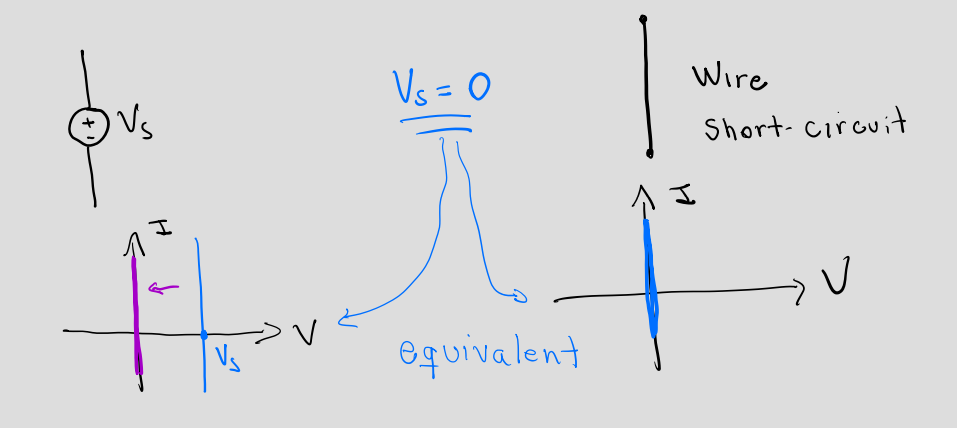$V_S = 0$ means that it allows any current to go through, however the voltage drop always remains zero. This is exactly what a wire element (sometimes called a short circuit) does.

### Open-circuit voltage: #

Voltage $V_\text{open} \equiv V_{Th}$ when $I_S = 0$. Used for thevenin equivalent

If we plot the $I$-$V$ graph of a current source $I_S$, we get the a horizontal line: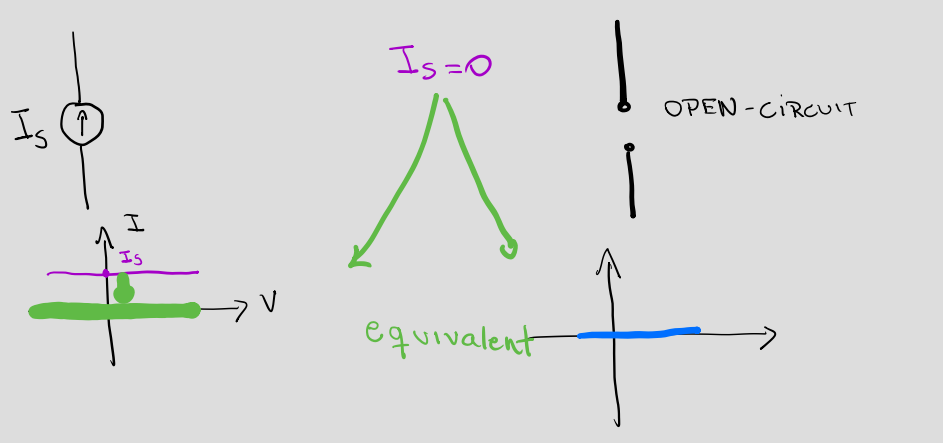If we turn off the current source, $I_S = 0$, which means no matter what voltage you apply, there will be no current. This is equivalent to an open circuit.

### Motivation #

When we add a new component to a circuit, it interacts through only 2 parameters: current $I$ and voltage $V$. Equivalent circuits are used to simplify interactions between circuits.

Let’s take the simplest case where interactions are only through one pair of nodes. In that case, we just have two possible quantities: the voltage across the nodes and the current flowing through the connections. The relationship between this current and this voltage would then fully define the interactions between the circuits. This is where the idea of equivalence comes in. If we have a circuit that exhibits the same $I$−$V$ relationship from the standpoint of a pair of nodes, the other circuit (the one you are interacting with) can’t tell the difference.

The idea of equivalence is to be able to replace one (or both) of the interacting circuits with a simpler circuit that will give us the same overall behavior.

### Limitations #

• The $I$-$V$ characteristic is the only feature preserved by an equivalent circuit
• Equivalence tells us nothing about the power in a circuit.
• From the standpoint of any other nodes in the circuit (i.e. any pairs of nodes), the circuit may or may not be equivalent
• That is, looking at the same circuit but examining a different pair of terminals may not produce equivalent $I$−$V$ relationship.

## Series & Parallel #

• These are proven starting page 10 of notes
• We cannot use these to simplify our circuit if there are dependent sources. Remember that only independent sources are zeroed out, and there are no resistor formulas for dependent sources. In addition, some resistor configurations cannot be decomposed into combinations of parallel and series resistances.
• Series:
• The voltage is the sum of the voltage drops of the individual components: $$V = V_1 + \dots + V_n = I (R_\text{total})$$
• From KCL we know elements will have the exact same current through them: $$I = I_1 = \dots = I_n$$
• Resistance is the sum of their individual resistances: $$R_\text{total} = R_1 + \dots R_n$$
• Parallel:
• From KVL we know elements will have the exact same voltage across them: $$V = V_1 = \dots = V_n$$
• The current in each individual element is found by Ohm’s law: $$I = I_1 + \dots + I_n = V R_\text{total}$$
• Total resistance will always be less than the value of the smallest resistance $$\frac{1}{R_\text{total}} = \sum_{i=1}^n \frac{1}{R_i}$$

Notation: We use $\parallel$ to denote parallel components

Comparison of effective resistance (left), inductance (middle) and capacitance (right) of two resistors, inductors and capacitors in series and parallel

## Thevenin #

Any linear two-terminal circuit can be replaced at terminals $A$–$B$ by an equivalent combination of a voltage source $V_{Th}$ in a series connection with a resistance $R_{Th}$ – wiki

1. Find $V_{Th}$:
• We recognize that it equals the difference in node potentials at $A$ and $B$, so performing NVA on the original circuit will yield $V_{Th}$
• Connect an open circuit across the two output terminals and measure the voltage across them. This measured $V_{oc}$ equals $V_{Th}$.
2. Find $R_{Th}$: Zero out any independent sources. Remember, this means voltage sources turn into a wire and current sources turn into an open circuit. Then apply either a test current into the terminal and measure the resultant voltage, or apply a test voltage and measure the resultant current. $R_{Th}= \frac{V_{test}}{I_{test}}$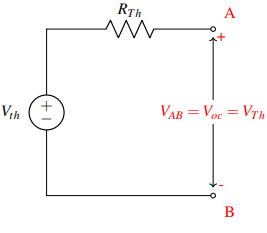## Norton #

Any linear two-terminal circuit can be replaced by a current source $I_{No}$ and a single resistor $R_{No}$ in parallel – wiki

1. Find $I_{No}$: Connect a short circuit across the two output terminals and measure the current through it. This measured $I_{Sc}$ equals $I_{No}$.
2. Find $R_{No}$: Zero out any independent sources. Remember, this means voltage sources turn into a short circuit and current sources turn into an open circuit. Then apply either a test current into the terminal and measure the resultant voltage, or apply a test voltage and measure the resultant current. $R_{Th}= \frac{V_{test}}{I_{test}}$
• Note that the second step doesn’t change because $R_{No}$ is equal to $R_{Th}$!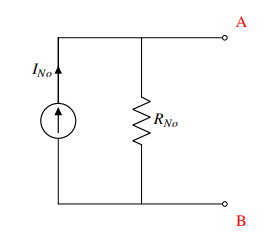### Relation / Conversion #

A Norton equivalent circuit is related to the Thévenin equivalent by

$$R = R_{Th} = R_{No}$$ $$V_{Th} = I_{No}R$$ $$I_{No} = \frac{V_{Th}}{R}$$Note that $R_{No}$ is equal to $R_{Th}$, since the slope of the $I$-$V$ curve is the same. Now, instead of looking at the $V$-axis intercept, we find the intersection with the $I$-axis: At the intersection with the $I$-axis, the voltage drop between $A$ and $B$ is zero, which is equivalent to placing a wire between $A$ and $B$ (i.e. shorting $A$ and $B$). We denote the current through the wire be $I_{No}$.

Why this works:

• Since the $I$-$V$ relationship is linear, we can calculate the slope (which is the reciprocal of resistance) from any two points. $V_{Th}$ and $I_{No}$ are the points where the $I$-$V$ curve crosses the$V$ and $I$ axes, respectively (see the left-hand figure).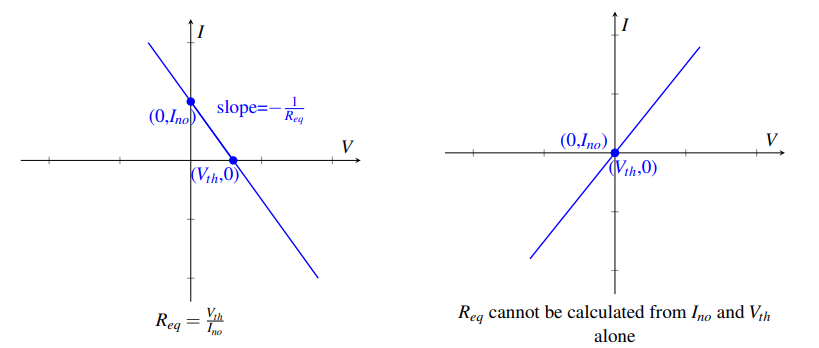• However, this method does not work if $V_{Th}$ and $I_{No}$ do not provide two unique points on the $I$-$V$ curve (see the right-hand figure).
• Specifically, this method only works if there is at least one independent source in the circuit.
• When there are no independent sources, $V_{Th} = I_{No} = 0$ which does not provide enough information to calculate Req.

## Superposition #

Recall two weeks back: To solve for the currents and node potentials in a circuit, we set up a matrix problem of the form $A\vec x =\vec b$ where $\vec x$ contained the unknown currents and node potentials, $\vec b$ contained the independent current and voltage sources, and $A$ described the relationship between them. Since this matrix equation describes a real system, we know that there is a unique solution. Therefore, $A$ is invertible: $$\vec x = A^{−1} \vec b$$ This means that we can describe any current or node potential (ie. any element of $\vec x$) as a linear combination of the independent current and voltage sources (the elements of $\vec b$).

For example, consider a circuit with $n$ independent sources voltage sources $V_{s1} \dots V_{sn}$, and $m$ independent current sources $I_{s1} \dots I_{sm}$. An arbitrary node potential $u_i$ (or equivalently, an arbitrary current $i_i$) can be written as $$u_i = \alpha_1 V_{s1} + \dots +\alpha_n V_{sn} + \beta_1 I_{s1} + \dots \beta_m I_{sm}$$ where the $\alpha$’s and $\beta$’s are coefficients from inverting $A$. Since this equation is linear, we can calculate each term of this equation separately and then add them together at the end.

For example, if we want to calculate the first term, $\alpha_1 V_{s1}$ we can set all of the other voltage and current sources to zero, then solve for $u_i$. Repeating this for every source then adding the results is equivalent to calculating $u_i$ with all of the sources present. However, splitting up the calculations can help us see simplifications and patterns that might be less obvious with all of the sources present.

TL;DR:

• For each independent source $k$ (either voltage source or current source)
• Set all other independent sources to $0$
• Voltage source: replace with a wire – no voltage drop
• Current source: replace with an open circuit – no current flows
• Compute the circuit voltages and currents due to this source $k$
• Compute $V_\text{out}$ by summing the $V_{\text{out; }k }$s for all $k$.

We can apply the idea of replacing elements with equivalent elements (e.g. replacing a $V_S = 0$ voltage source with a wire) to resistors as well. When do resistors have an equivalent representation? Recall that by Ohm’s law, the $I$-$V$ graph across a resistor. From this we can see:

• Zero voltage source and zero resistance are equivalent to wires (i.e. short circuits);
• Zero current source and infinite resistance are equivalent to open circuits